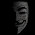Finite State Machine (FSM) Coding In Verilog

There is a special Coding style for State Machines in VHDL as well as in Verilog. Let us consider below given state machine which is a “1011” overlapping sequence detector. Output becomes ‘1’ when sequence is detected in state S4 else it remains ‘0’ for other states.
Verilog Code for FSM:
// 4-State Moore state machine
// A Moore machine's outputs are dependent only on the current state.
// The output is written only when the state changes.  (State
// transitions are synchronous.)
module seq_dect
(
input    clk, data_in, reset,
output reg  data_out
);
// Declare state register
reg        [2:0]state;
// Declare states
parameter S0 = 0, S1 = 1, S2 = 2, S3 = 3, S4 = 4;

// Determine the next state
always @ (posedge clk or posedge reset) begin
if (reset)
state <= S0;
else
case (state)
S0:
if (data_in)
state <= S1;
else
state <= S0;
S1:
if (data_in)
state <= S1;
else
state <= S2;
S2:
if (data_in)
state <= S3;
else
state <= S2;
S3:
if (data_in)
state <= S4;
else
state <= S2;
S4:
if (data_in)
state <= S1;
else
state <= S2;
endcase // case (state)
end // always @ (posedge clk or posedge reset)
// Output depends only on the state
always @ (state) begin
case (state)
S0:
data_out = 1'b0;
S1:
data_out = 1'b1;
S2:
data_out = 1'b0;
S3:
data_out = 1'b1;
S4:
data_out = 1'b1;
default:
data_out = 1'b0;
endcase // case (state)
end // always @ (state)

endmodule // moore_mac

1.1.